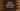# Python program to find factors of a number# Find factors of a number in python :

In this tutorial, we will learn how to find the factors of a number in python. The program will ask the user to enter a number. It will then print out the factors for that number. For example, if the number is 12, it will print 1,2,3,4,6,12 as the output.

## Algorithm to use :

The following algorithm we will use to solve this problem :

1. Ask the user to enter a number. Read it and store it in a variable.
2. Using one loop, check for all numbers if it is a divisor or not starting from 1 to the user-provided number.
3. If any number is a divisor, print out the number.
4. Exit the program.

As you can see, we will use one loop to print out the factors of a number. We will show you to solve this problem by using a for loop and while loop. Both approaches will give the same output.

## Python program to find factors of a number using for loop :

Let’s try to find out the factors using a for loop :

``````#1
def print_factors(n):
#2
for i in range(1, n+1):
#3
if n % i == 0:
print(i)

#4
number = int(input("Enter a number : "))

#5
print("The factors for {} are : ".format(number))
print_factors(number)``````

### Explanation :

The commented numbers in the above program denote the step numbers below :

1. Create one method printfactors_ to find out all factors of a number. This function takes one integer value as a parameter.
2. Using one for loop, iterate over all numbers from 1 to n.
3. Check for each number in the loop if it is a divisor of the given number or not. If yes, print out the number.
4. This is the actual start point of the program. Ask the user to enter a number. Read the input value as an integer and store it in variable number.
5. Print out the factors for that number using the print_factors method.

### Sample output :

``````Enter a number : 12
The factors for 12 are :
1
2
3
4
6
12

Enter a number : 20
The factors for 20 are :
1
2
4
5
10
20``````

## Python program to find factors of a number using while loop :

Now, let’s try to find out the factors using a while loop :

``````def print_factors(n):
i = 1
while(i < n+1):
if n % i == 0:
print(i)
i = i + 1

number = int(input("Enter a number : "))

print("The factors for {} are : ".format(number))
print_factors(number)``````

### Explanation :

As you can see, only the print_factors method is different from this approach.

1. Initialize one variable* i* as 1 at the start of this method.
2. Using one while loop, iterate till* i* is less than* n+1.*
3. Check if the current value is a divisor of n or not. If yes, print out the value.
4. Increment the value of i.

### Output :

``````Enter a number : 21
The factors for 21 are :
1
3
7
21

Enter a number : 15
The factors for 15 are :
1
3
5
15``````

The above example programs are available on Github.

### Conclusion :

As you can see that using a for loop or while loop, we can find out the factors of a number. Try to run both programs and drop one comment below if you have any queries.